# playAnimation

Play animation objects in a MATLAB figure window

## Syntax

``playAnimation``
``playAnimation(fig)``
``playAnimation(___,Name,Value)``

## Description

example

````playAnimation` plays animation objects in a MATLAB® figure window. The animation objects must be created using the `fanimator` function.By default, the variable `t = sym('t')` is the time parameter of the animation objects. `playAnimation` plays the animation with 10 frames per unit interval of `t` within the range of `t` from 0 to 10.```

example

````playAnimation(fig)` plays animation objects in the figure `fig`.```

example

````playAnimation(___,Name,Value)` plays the animation objects with the specified `Name,Value` pair arguments. Use this option with any of the input argument combinations in the previous syntaxes.```

## Examples

collapse all

First, create an animation object of a moving circle using `fanimator`.

Create two symbolic variables, `t` and `x`. The variable `t` defines the time parameter of the animation. Use `t` to set the center of the circle at `(t,1)` and `x` to parameterize the perimeter of the circle within the range `[-pi pi]`. Set the x-axis and y-axis to be equal length.

```syms t x fanimator(@fplot,cos(x)+t,sin(x)+1,[-pi pi]) axis equal ```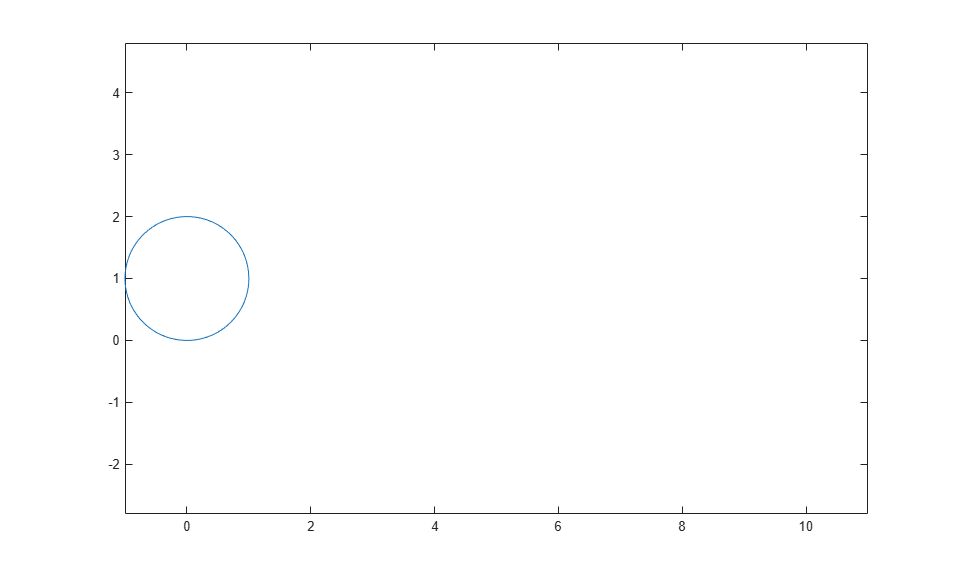Next, enter the command `playAnimation` to play the animation.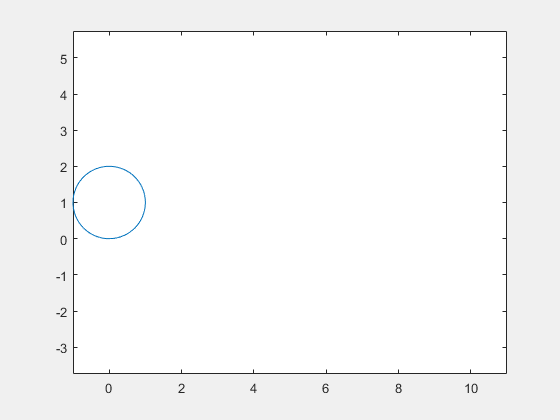By default, `playAnimation` plays an animation with 10 generated frames per unit time within the range of `t` from 0 to 10.

Create two symbolic variables, `t` and `x`. The variable `t` defines the time parameter of the animation.

```syms t x ```

Create a circle animation object using `fanimator`. Use `t` to set the center of the circle at `(t,1)` and `x` to parameterize the perimeter of the circle within the range `[-pi pi]`. Set the x-axis and y-axis to be equal length.

```fanimator(@fplot,cos(x)+t,sin(x)+1,[-pi pi]) axis equal ```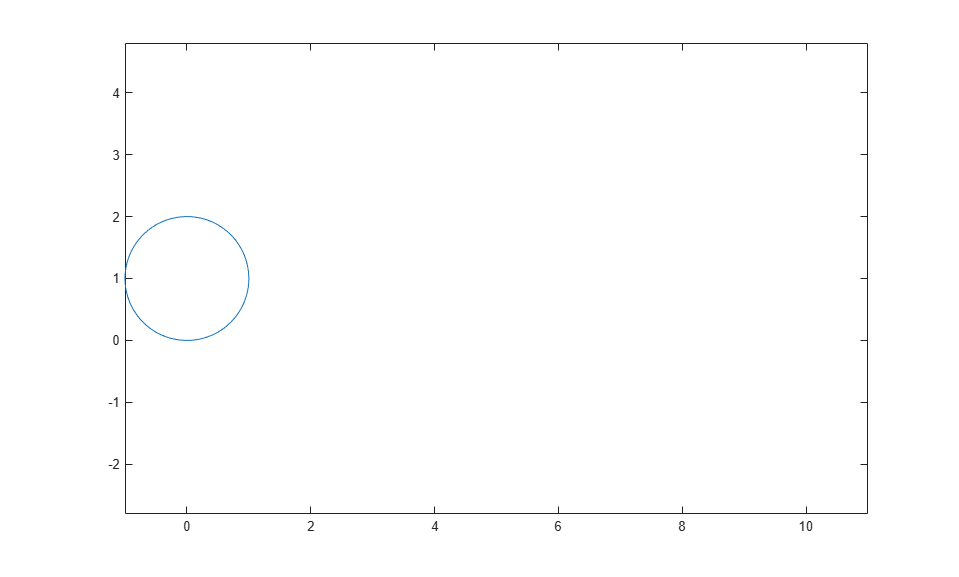Add a piece of text to count the elapsed time by using the `text` function. Use `num2str` to convert the time parameter to a string.

```hold on fanimator(@(t) text(9,3,"Timer: "+num2str(t,2))) hold off ```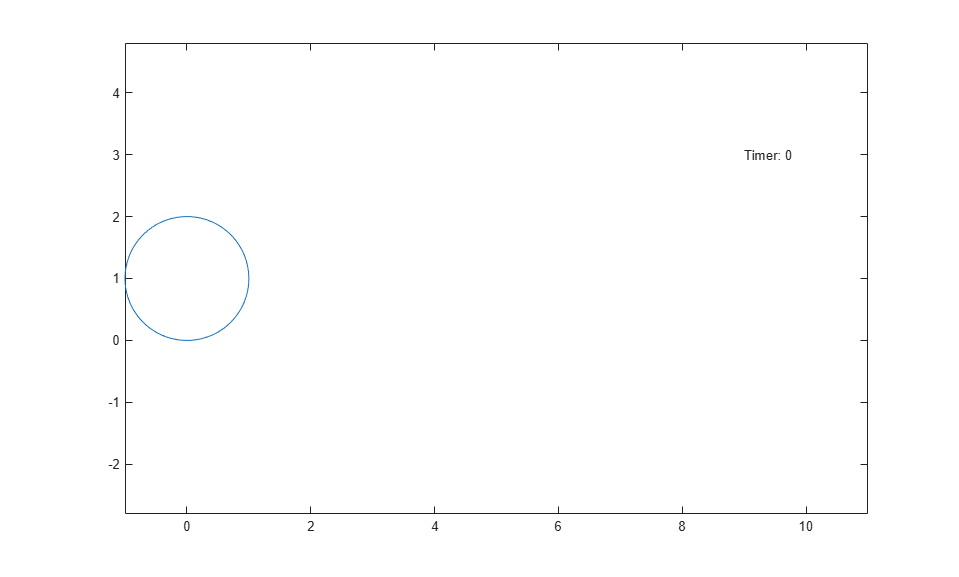By default, `playAnimation` plays the animation with 10 generated frames per unit time within the range of `t` from 0 to 10. Change the range of the time parameter to `[4 8]` using the `'AnimationRange'` property. Change the frame rate per unit time to 4 using the `'FrameRate'` property. Play the animation in the current figure by entering the following command.

`playAnimation(gcf,'AnimationRange',[4 8],'FrameRate',4)`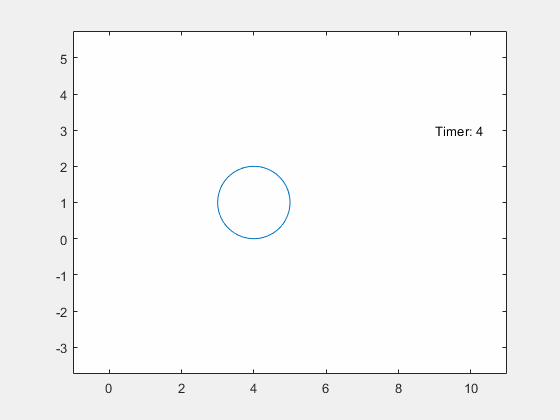Create a UI figure. Specify the UI axes of the figure.

```fig = uifigure; ax = uiaxes(fig);```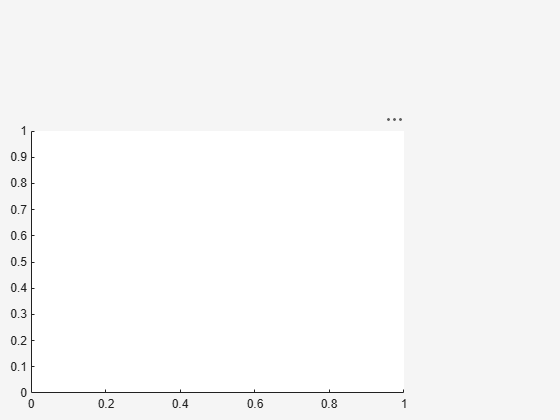Add an animation object to the UI axes using `fanimator`. Create two symbolic variables, `x` and `t`. Plot a curve that grows exponentially as a function of time `t` within the interval `[0 3]`.

```syms x t; fanimator(ax,@fplot,exp(x),[0 t],'r','AnimationRange',[0 3])```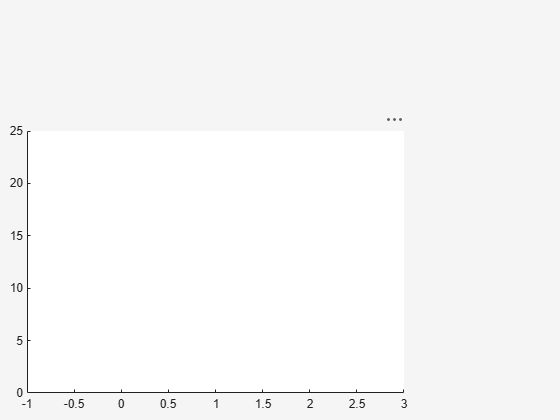Play the animation in the UI figure `fig` by entering the command `playAnimation(fig)`. Alternatively, you can also use the command `playAnimation(ax.Parent)`.## Input Arguments

collapse all

Target figure, specified as a `Figure` object. For more information about `Figure` objects, see `figure`.

### Name-Value Arguments

Specify optional pairs of arguments as `Name1=Value1,...,NameN=ValueN`, where `Name` is the argument name and `Value` is the corresponding value. Name-value arguments must appear after other arguments, but the order of the pairs does not matter.

Before R2021a, use commas to separate each name and value, and enclose `Name` in quotes.

Example: `'Backwards',true,'FrameRate',25`

Range of the animation time parameter, specified as a two-element row vector. The two elements must be real values that are increasing.

Example: `[-2 4.5]`

Frame rate, specified as a positive value. The frame rate defines the number of frames per unit time when playing the animation objects.

Example: `30`

Backward option, specified as a logical value (boolean). If you specify the option `true`, then the function plays the animation backwards.

Example: `true`

Speed factor, specified as a real nonzero value. The speed factor sets the ratio of one unit interval of the animation time parameter to one second of clock time.

• If you specify a negative value for `'SpeedFactor'` and keep the default value `0` (`false`) for `'Backwards'` option, then the function plays the animation backwards with the specified speed factor. For example, `playAnimation('SpeedFactor',-1)` launches the same animation as `playAnimation('Backwards',true)`.

• If you specify a zero value for `'SpeedFactor'`, then `playAnimation('SpeedFactor',0)` launches a still frame indefinitely and does not play any animation.

Example: `2`

## Tips

• When you create a graph by using a plotting function, such as `fplot`, MATLAB creates a series of graphics objects. You can then animate a specific property of the graphics objects by using the `fanimator` and the `playAnimation` functions. Note that some functions, such as `title` and `xlabel`, create text objects that cannot be animated. Instead, use the `text` function to create text objects that can be animated.

## Version History

Introduced in R2019a